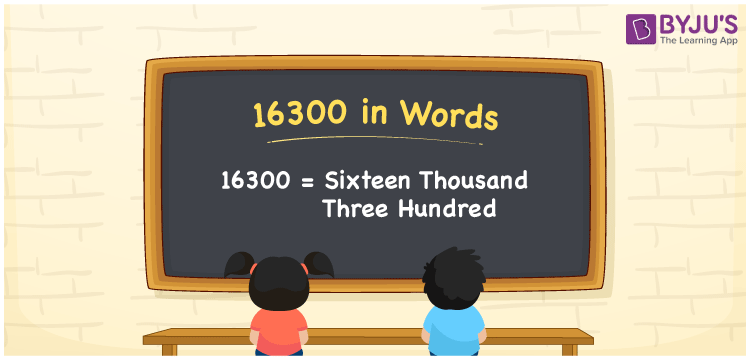# 16300 in Words

16300 in wordsis denoted by Sixteen Thousand Three Hundred. For instance, if you earned Rs. 16300 per month, you can say, “I earned Rupees Sixteen Thousand Three Hundred per month”. 16300 is a cardinal number since it expresses the values. In this article, we will discuss the procedure of how to write number 16300 in English words.

 16300 in Words Sixteen Thousand Three Hundred Sixteen Thousand Three Hundred in numerical form 16300

## 16300 in English Words

In Mathematics, we generally, write numbers in words with the help of the English alphabet. Therefore, we can spell the number 16300 in English as Sixteen Thousand Three Hundred.## How to Write 16300 in Words?

To convert the number 16300, we use a place value system. The following table shows the place value chart for the number 16300.

 Ten Thousands Thousands Hundreds Tens Ones 1 6 3 0 0

Hence, we can write the expanded form as:

1 x Ten Thousand + 6 x Thousand + 3 x Hundred + 0 x Ten + 0 x One

= 1 x 10000 + 6 x 1000 + 3 x 100 + 0 x 10 + 0 x 1

= 10000 + 6000 + 300 + 0 + 0

= 10000 + 6000 + 300

= 16300

= Sixteen Thousand Three Hundred

Therefore, 16300 in words is written as Sixteen Thousand Three Hundred

Interesting way of writing 16300 in words

1 = One

16 = Sixteen

163 = One Hundred and Sixty-Three

1630 = One Thousand Six Hundred Thirty

16300 = Sixteen Thousand Three Hundred

Thus, the word form of the number 16300 is Sixteen Thousand Three Hundred

16300 is a natural number that is one less than 16301 and one greater than 16299

• 16300 in words – Sixteen Thousand Three Hundred
• Is 16300 an odd number? – No
• Is 16300 an even number? – Yes
• Is 16300 a perfect square number? – No
• Is 16300 a perfect cube number? – No
• Is 16300 a prime number? – No
• Is 16300 a composite number? – Yes

## Frequently Asked Questions on 16300 in Words

Q1

### How to write 16300 in words?

We can write16300 in words as Sixteen Thousand Three Hundred.
Q2

### Simplify 15000 + 1300, and express in words.

Simplifying 15000 + 1300, we get 16300. Therefore, the number 16300 in words is Sixteen Thousand Three Hundred.
Q3

### Is 16300 a composite number?

Yes, 16300 is a composite number.